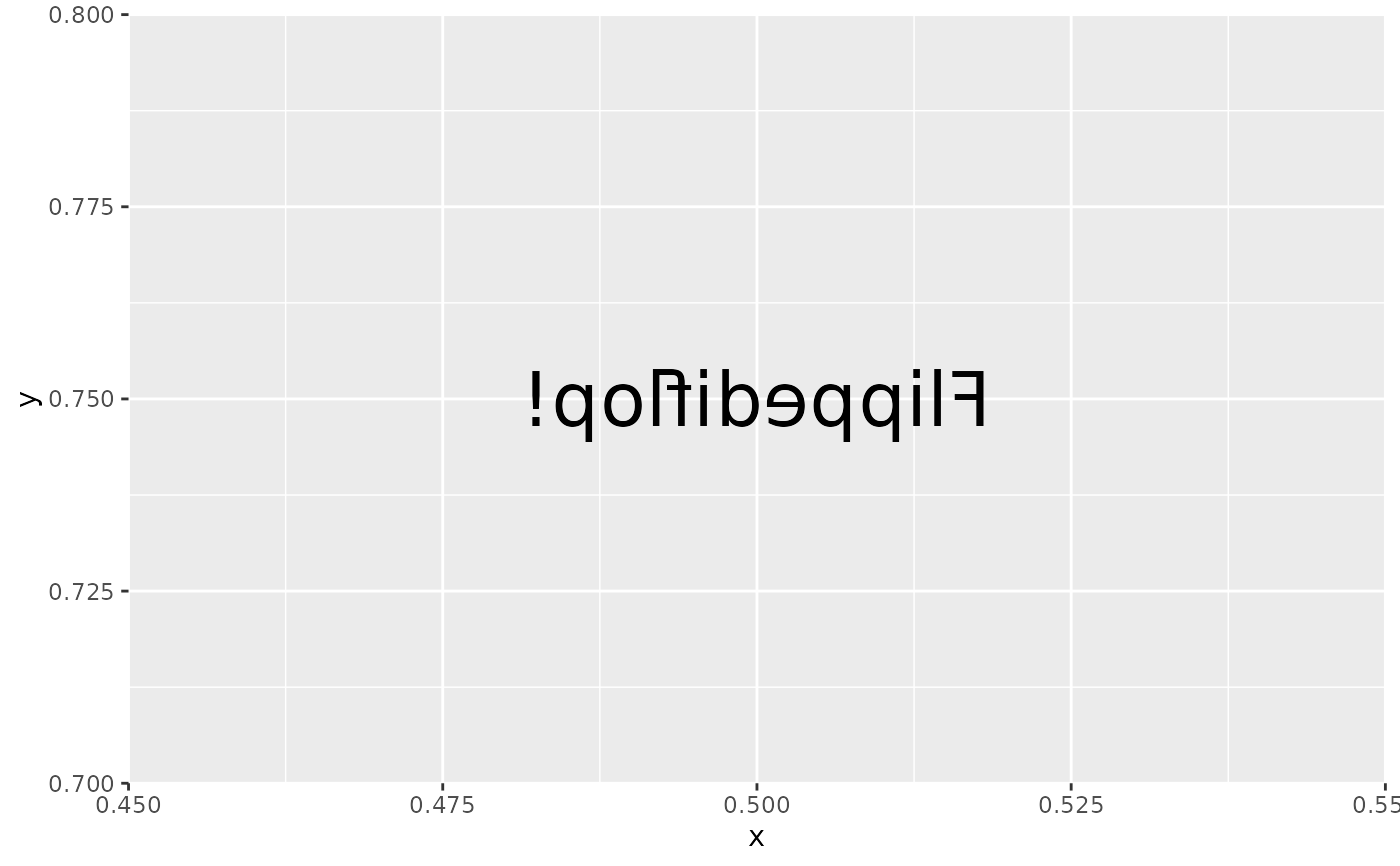These utility functions can help when creating custom filters (using with_custom()) as they can provide information about the current rendering context.

viewport_location()

index_raster(raster, cols, rows)

get_raster_area(raster, xmin, ymin, xmax, ymax)

set_raster_area(raster, value, xmin, ymin)

get_viewport_area(raster)

set_viewport_area(raster, value)

viewport_is_clipping()

current_resolution()

to_pixels(x, y_axis = FALSE, location = FALSE)

from_pixels(x)

## Arguments

raster

A raster or nativeRaster object

cols, rows

Column and row indices

xmin, ymin, xmax, ymax

Boundaries of the area in pixels. 0,0 is the top-left corner

value

An object of the same type as raster

x

A numeric or unit object

y_axis

is the unit pertaining to the y-axis? Defaults to FALSE (i.e. it is measured on the x-axis)

location

is the unit encoding a location? Defaults to FALSE (i.e. it is encoding a dimension). Pixel locations are encoded based on a top-left starting point, as opposed to grid's bottom-left coordinate system. This means that y-axis locations will flip around when converted to pixels.

## Value

Depends on the function - see details.

## Details

• viewport_location(): Returns the bounding box defining the current viewport in pixels in the order xmin, ymin, xmax, ymax

• index_raster(): Is a version of the classic [,] indexing that is aware of the row-major order of rasters

• get_raster_area(): Extracts an area of a raster based on a bounding box

• set_raster_area(): Sets an area of a raster to a new raster value

• get_viewport_area(): A version of get_raster_area() that specifically extract the area defined by the current viewport

• set_viewport_area(): A version of set_raster_area() that specifically sets the area defined by the current viewport

• viewport_is_clipping(): Returns TRUE if the current viewport has clipping turned on

• current_resolution(): Returns the resolution of the active device in ppi (pixels-per-inch)

• to_pixels(x): Converts x to pixels if x is given as a unit object. It is assumed that x encodes a dimension and not a location. If x is a numeric it is assumed to already be in pixels

• from_pixels: Converts a numeric giving some pixel dimension to a unit object.

## Examples

# These functions are intended to be used inside filter functions, e.g.
library(ggplot2)

flip_raster <- function(raster, horizontal = TRUE) {
# Get the viewport area of the raster
vp <- get_viewport_area(raster)

# Get the columns and rows of the raster - reverse order depending on
# the value of horizontal
dims <- dim(vp)
rows <- seq_len(dims)
cols <- seq_len(dims)
if (horizontal) {
cols <- rev(cols)
} else {
rows <- rev(rows)
}

# change the order of columns or rows in the viewport raster
vp <- index_raster(vp, cols, rows)

# Assign the modified viewport back
set_viewport_area(raster, vp)
}

ggplot() +
with_custom(
geom_text(aes(0.5, 0.75, label = 'Flippediflop!'), size = 10),
filter = flip_raster,
horizontal = TRUE
)